Choose Language Hide Translation Bar
Highlighted

## Is there a way to display a Dynamic X-Reference Line across multiple plots?

Hi,

I am using Graph Builder to display:

•        the number of defects within a batch as parts are processed through a certain manufacturing tool    TOP PLOT
•        the E10 Equipment State changes the tool went through during the same time period                       CENTER PLOT
•       a process monitor taken from test substrates also processed during the same  period.                       BOTTOM PLOT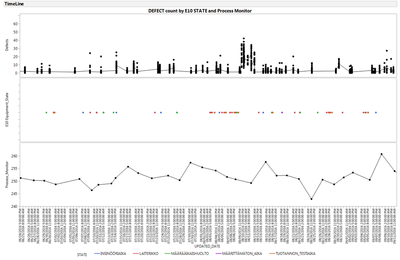The "updated_date" is the only common factor between all data sets, which have been collected from 3 different databases

I would like to have the possibility to have an X Reference line displayed while I click and hold the mouse on any of  the plots. On mouse release it should disappear.

The Reference line should  be displayed across all plots simultaneously.

An example of what I need  is seen below: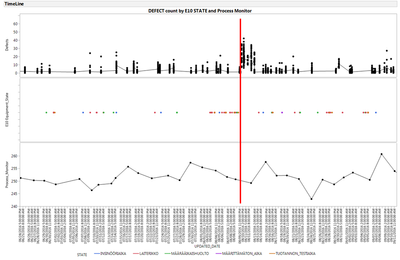Looking forward to seeing what creative solutions may be provided

- Philip

1 ACCEPTED SOLUTION

Accepted Solutions
Highlighted

## Re: Is there a way to display a Dynamic X-Reference Line across multiple plots?

Using the original method, your code would have to update 2 AND 3 when 1 is manipulated (and cyclic permutations thereof).

But with Craige's '<<inval' approach, the code becomes much simpler:

Names Default To Here( 1 );

dt = Open( "\$SAMPLE_DATA/Big Class.jmp" );

gb = dt << Graph Builder(

Size( 528, 448 ),

Show Control Panel( 0 ),

Variables( X( Transform Column( "Row", Formula( Row() ) ) ), Y( :height ), Y( :weight ), Y( :age ) ),

);

::exx = 1;

gbfb3 = Report( gb )[FrameBox( 3 )];

Pen Color( "Red" );

Pen Size( 2 );

Mousetrap(

::exx = x;

gb << inval;

);

V Line( ::exx );

);

gbfb1 = Report( gb )[FrameBox( 1 )];

Pen Color( "Red" );

Pen Size( 2 );

Mousetrap(

::exx = x;

gb << inval;

);

V Line( ::exx );

);

gbfb2 = Report( gb )[FrameBox( 2 )];

Pen Color( "Red" );

Pen Size( 2 );

Mousetrap(

::exx = x;

gb << inval;

);

V Line( ::exx );

);

5 REPLIES 5
Highlighted

## Re: Is there a way to display a Dynamic X-Reference Line across multiple plots?

Names Default To Here( 1 );

dt = Open( "\$SAMPLE_DATA/Big Class.jmp" );

gb = dt << Graph Builder(

Size( 528, 448 ),

Show Control Panel( 0 ),

Variables( X( Transform Column( "Row", Formula( Row() ) ) ), Y( :height ), Y( :weight ) )

);

::exx = 1;

gbfb1 = Report(gb)[FrameBox(1)];

gs1 = Expr(

PenColor("Red");

PenSize(2);

Mousetrap(::exx = x; gbfb2 << removeGraphicsScript(1); gs2);

VLine(::exx);

);

);

gbfb2 = Report(gb)[FrameBox(2)];

gs2 = Expr(

PenColor("Red");

PenSize(2);

Mousetrap(::exx = x; gbfb1 << removeGraphicsScript(1); gs1);

VLine(::exx);

);

);

gs1;

gs2;

Highlighted

## Re: Is there a way to display a Dynamic X-Reference Line across multiple plots?

Hi Ian,

Thank you for  your fast response.

This is exactly what I am looking for but I need it to work across 3 plots

Using the "\$SAMPLE_DATA/Big Class.jmp dataset I added a plot for :age but unfortunately when I try to set this up to work across 3 plots I have been unable to get it working. I can get 2 out of 3 working at any time.

Would it be possible to add some comments to the script to explain what is the logic? My apologies for this inconvenience.

In my case it would suffice that when  clicking on the center plot (:weight) the reference line would be drawn upwards and downwards covering all plots.

Here is what I have done:

Names Default To Here( 1 );

dt = Open( "\$SAMPLE_DATA/Big Class.jmp" );

gb = dt << Graph Builder(

Size( 528, 448 ),

Show Control Panel( 0 ),

Variables( X( Transform Column( "Row", Formula( Row() ) ) ), Y( :height ), Y( :weight ), Y( :age ) ),

);

::exx = 1;

gbfb3 = Report(gb)[FrameBox(3)];

gs3 = Expr(

PenColor("Red");

PenSize(2);

Mousetrap(::exx = x; gbfb1 << removeGraphicsScript(1); gs1);

VLine(::exx);

);

);

gbfb1 = Report(gb)[FrameBox(1)];

gs1 = Expr(

PenColor("Red");

PenSize(2);

Mousetrap(::exx = x; gbfb2 << removeGraphicsScript(1); gs2);

VLine(::exx);

);

);

gbfb2 = Report(gb)[FrameBox(2)];

gs2 = Expr(

PenColor("Red");

PenSize(2);

Mousetrap(::exx = x; gbfb3 << removeGraphicsScript(1); gs3);

VLine(::exx);

);

);

gs1;

gs2;

gs3;

The resulting plot looks like this: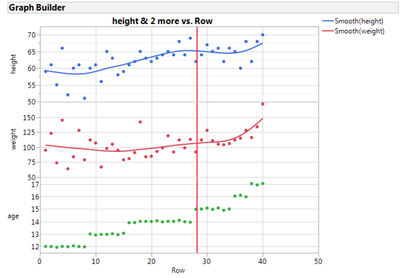When I click inside the "age" plot, all goes well with the Ref. Line moving with the mouse across all plots.

However when I click inside the "height" plot  the following happens:

The Reference line moves through "height" and "weight" but not "age"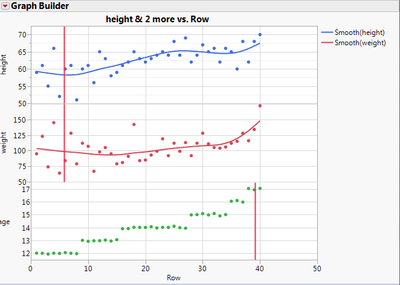Likewise when I click inside the "weight" plot  the following happens:

The Reference line moves through "weight" and "age" but not "height"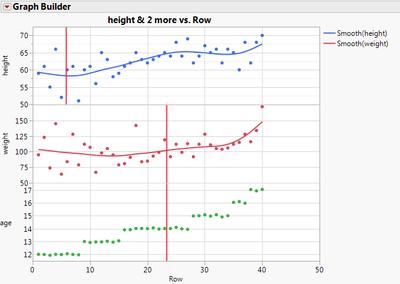Could you please take a look?

Thanks,

Philip

Highlighted

## Re: Is there a way to display a Dynamic X-Reference Line across multiple plots?

Using the original method, your code would have to update 2 AND 3 when 1 is manipulated (and cyclic permutations thereof).

But with Craige's '<<inval' approach, the code becomes much simpler:

Names Default To Here( 1 );

dt = Open( "\$SAMPLE_DATA/Big Class.jmp" );

gb = dt << Graph Builder(

Size( 528, 448 ),

Show Control Panel( 0 ),

Variables( X( Transform Column( "Row", Formula( Row() ) ) ), Y( :height ), Y( :weight ), Y( :age ) ),

);

::exx = 1;

gbfb3 = Report( gb )[FrameBox( 3 )];

Pen Color( "Red" );

Pen Size( 2 );

Mousetrap(

::exx = x;

gb << inval;

);

V Line( ::exx );

);

gbfb1 = Report( gb )[FrameBox( 1 )];

Pen Color( "Red" );

Pen Size( 2 );

Mousetrap(

::exx = x;

gb << inval;

);

V Line( ::exx );

);

gbfb2 = Report( gb )[FrameBox( 2 )];

Pen Color( "Red" );

Pen Size( 2 );

Mousetrap(

::exx = x;

gb << inval;

);

V Line( ::exx );

);

Highlighted

## Re: Is there a way to display a Dynamic X-Reference Line across multiple plots?

Each cell in the trellis plot that Graph Builder produces is a separate 'FrameBox()', and the challenge is to update each of these when only one is changed. The code above relies on repeatedly deleting and reinstating a graphics script for each FrameBox(), and this is clumsy to say the least.

Craige@jmp was kind enough to point out a better way: Simply send the Graph Builder object itself an '<< inval' message, which forces all of the associated FrameBoxes to redraw appropriately:

Names Default To Here( 1 );

dt = Open( "\$SAMPLE_DATA/Big Class.jmp" );

gb = dt << Graph Builder(

Size( 528, 448 ),

Show Control Panel( 0 ),

Variables( X( Transform Column( "Row", Formula( Row() ) ) ), Y( :height ), Y( :weight ) )

);

::exx = 1;

gbfb1 = Report( gb )[FrameBox( 1 )];

Pen Color( "Red" );

Pen Size( 2 );

Mousetrap(

::exx = x;

gb << inval; // make sure the other graph updates

);

V Line( ::exx );

);

gbfb2 = Report( gb )[FrameBox( 2 )];

Pen Color( "Red" );

Pen Size( 2 );

Mousetrap(

::exx = x;

gb << inval; // make sure the other graph updates

);

V Line( ::exx );

);

Highlighted

## Re: Is there a way to display a Dynamic X-Reference Line across multiple plots?

Thank you Ian and Craig for your solution(s).

This works beautifully

If you are up to another challenge, .....  I was wondering if activated labels could be displayed when the Reference Line is placed on any point in any chart.

Recall that there will never be a situation that points will occur exactly on the same x-axis location in my application.

I my case, the labels would be operator written comments relating to testing, manufacturing or process monitoring.

This is more "nice to have" rather than necessary but it would be very nice

- Philip

Article Labels

There are no labels assigned to this post.